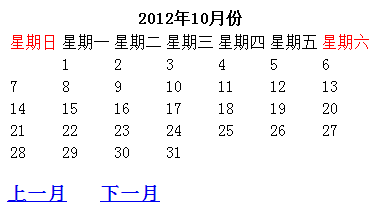# PHP制作万年历

更新时间：2015年01月07日 16:32:27   投稿：hebedich我要评论

\$days的作用:知道要处理的月份共有多少天，就可以通过循环输出天数了

\$dayofweek的作用:只有知道每个月的1号是星期几，才能知道在输出天数之前需要输出多少空格(空白)“万年历类”的代码如下：

<?php
/**
* PHP万年历
* @author Fly 2012/10/16
*/
class Calendar{
protected \$_table;//table表格
protected \$_currentDate;//当前日期
protected \$_year;    //年
protected \$_month;    //月
protected \$_days;    //给定的月份应有的天数
protected \$_dayofweek;//给定月份的 1号 是星期几
/**
* 构造函数
*/
public function __construct()
{
\$this->_table="";
\$this->_year  = isset(\$_GET["y"])?\$_GET["y"]:date("Y");
\$this->_month = isset(\$_GET["m"])?\$_GET["m"]:date("m");
if (\$this->_month>12){//处理出现月份大于12的情况
\$this->_month=1;
\$this->_year++;
}
if (\$this->_month<1){//处理出现月份小于1的情况
\$this->_month=12;
\$this->_year--;
}
\$this->_currentDate = \$this->_year.'年'.\$this->_month.'月份';//当前得到的日期信息
\$this->_days           = date("t",mktime(0,0,0,\$this->_month,1,\$this->_year));//得到给定的月份应有的天数
\$this->_dayofweek    = date("w",mktime(0,0,0,\$this->_month,1,\$this->_year));//得到给定的月份的 1号 是星期几
}
/**
* 输出标题和表头信息
*/
protected function _showTitle()
{
\$this->_table.="<tbody><tr>";
\$this->_table .="<td style='color:red'>星期日</td>";
\$this->_table .="<td>星期一</td>";
\$this->_table .="<td>星期二</td>";
\$this->_table .="<td>星期三</td>";
\$this->_table .="<td>星期四</td>";
\$this->_table .="<td>星期五</td>";
\$this->_table .="<td style='color:red'>星期六</td>";
\$this->_table.="</tr>";
}
/**
* 输出日期信息
* 根据当前日期输出日期信息
*/
protected function _showDate()
{
\$nums=\$this->_dayofweek+1;
for (\$i=1;\$i<=\$this->_dayofweek;\$i++){//输出1号之前的空白日期
\$this->_table.="<td> </td>";
}
for (\$i=1;\$i<=\$this->_days;\$i++){//输出天数信息
if (\$nums%7==0){//换行处理：7个一行
\$this->_table.="<td>\$i</td></tr><tr>";
}else{
\$this->_table.="<td>\$i</td>";
}
\$nums++;
}
\$this->_table.="</tbody></table>";
\$this->_table.="<h3><a href='?y=".(\$this->_year)."&m=".(\$this->_month-1)."'>上一月</a>   ";
\$this->_table.="<a href='?y=".(\$this->_year)."&m=".(\$this->_month+1)."'>下一月</a></h3>";
}
/**
* 输出日历
*/
public function showCalendar()
{
\$this->_showTitle();
\$this->_showDate();
echo \$this->_table;
}
}
\$calc=new Calendar();
\$calc->showCalendar();